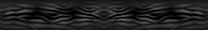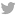• ### Thought of the Day

As kids in school, we spend our days in the winter cold,but as summer approaches, we just feel old.

Where We're Going by Kevin Graham

A Wrinkle in Time by Madeleine L’Engle

• ### 42

 Blabberbox » 42 » One Equals Zero# One Equals Zero

September 26th, 2006 | Posted by pftq in 42 | #

## Proving 1=0, 2=1, and so on…

Here is a pretty tricky proof that I’ve come across several times. It basically proves that a+b=a, which would mean that 1+0=0, 1=0, 2=1, and so on.

I know very well how to prove this wrong, but do you?a = b

ab = b²

ab - a² = b² - a²

a(b - a) = (b + a)(b - a)

a = b + a

If a and b are 1, then 1 = 1 + 1

1 = 2

0 = 1

1569 unique view(s)

#### Responses

1. george said,
Dec-29-2008, 11:56pm

no

2. Bobby said,
Mar-16-2009, 06:23pm

im probably a little late but at the part where a(b-a)=(b+a)(b-a)

since b=a
b-a=0 and if u multiply 0 each side you would end up with 0

3. dave said,
Mar-28-2009, 02:36pm

your dividing by zero.  you cant divide both sides by (b-a). dividing by zero is undefined

4. kenneth said,
Jan-02-2012, 08:03pm

a(b - a) = (b + a)(b - a)
-(b - a)         -(b - a)
a = (b + a)

Its not equal

5. kenneth said,
Jan-02-2012, 08:15pm

However

"0 IS 1 number" on the number scale.
In the begining is- 0 we now have 1 number. Oh its now 2. or is it three? Now im counting 4! ah <><>
Its called Axiom of infinity i think?

A war between what it is and what it means

6. Avinash said,
Feb-08-2014, 08:06am

niceEnter the code from image: